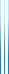# PublicationsManuscripts
Suppose $n$ points are thrown on the circumference of a unit-circumference circle, partitioning the circumference into $n$ segments. What is the expected length of the $k$th smallest segment? There is a well-known formula for this expectation.
We consider the expected length of $k$th smallest two adjacent segments. We develop a method for computing them exactly, and compute the expectations for several small $n,k$. The results do not seem to fit into a nice pattern.